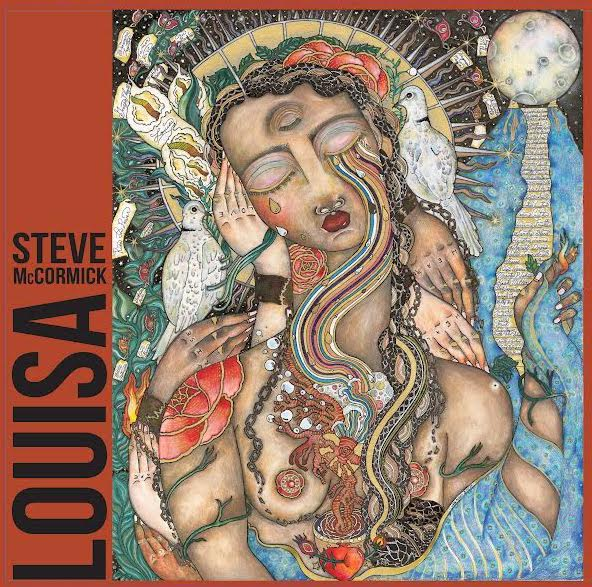Art, History, Music, Politics, and Spirituality For The Modern Alchemist – circulation in over 129 countries

# The Sign of Watatsumi Year 18,004 Begins: December 14th 2016

There are eight months in the Calendar of Mu, sometimes referred to as the Nyarzirian Calendar. Each month consists of forty-five days, and represents a system of the eight hexagrams moving through the five elements. The Ninzuwu invokes the mudra/mantra formulae associated with each hexagram that rules a particular day according to their place in the work. The chart below illustrates the daily Vasuh letters invoked and the ruling “deity” for the said hexagram:
1. December 14th 2016 = Midzuhanome = 3rd Hexagram = Shki-Zhee
2. December 15th 2016 = Susanoo = 5th Hexagram = Phe-Tuu-Nzu
3. December 16th 2016 = Nudzuwata = 8th Hexagram = Tuu-Zhee
4. December 17th 2016 = Watatsumi = 29th Hexagram = Aum-Shki
5. December 18th 2016 = Ugajin = 39th Hexagram = Phe-Bnhu-Shki
6. December 19th 2016 = Ame-no-Uzume = 48th Hexagram = Hmu-Shki-Aum
7. December 20th 2016 = Izanami-no-Mikoto = 60th Hexagram = Nzu-Phe-Shki
8. December 21st 2016 = Ajisukitakahikone = 63rd Hexagram = Aum-Nzu
9. December 22nd 2016 =Entering Earth Element
10. December 23rd 2016 = Midzuhanome = 3rd Hexagram = Shki-Zhee
11. December 24th 2016 = Susanoo = 5th Hexagram = Phe-Tuu-Nzu
12. December 25th 2016 = Nudzuwata = 8th Hexagram = Tuu-Zhee
13. December 26th 2016 = Watatsumi = 29th Hexagram = Aum-Shki
14. December 27th 2016 = Ugajin = 39th Hexagram = Phe-Bnhu-Shki
15. December 28th 2016 = Ame-no-Uzume = 48th Hexagram = Hmu-Shki-Aum
16. December 29th 2016 = Izanami-no-Mikoto = 60th Hexagram = Nzu-Phe-Shki
17. December 30th 2016 = Ajisukitakahikone = 63rd Hexagram = Aum-Nzu
18. December 31st 2016 =Entering Water Element
19. January 1st 2017 = Midzuhanome = 3rd Hexagram = Shki-Zhee
20. January 2nd 2017 = Susanoo = 5thHexagram = Phe-Tuu-Nzu
21. January 3rd 2017 = Nudzuwata = 8thHexagram = Tuu-Zhee
22. January 4th  2017 = Watatsumi = 29thHexagram = Aum-Shki
23. January 5th  2017 = Ugajin = 39thHexagram = Phe-Bnhu-Shki
24. January 6th  2017 = Ame-no-Uzume = 48thHexagram = Hmu-Shki-Aum
25. January 7th 2017 = Izanami-no-Mikoto = 60thHexagram = Nzu-Phe-Shki
26. January 8th 2017 = Ajisukitakahikone = 63rdHexagram = Aum-Nzu
27. January 9th 2017 =Entering Fire Element
28. January 10th 2017 = Midzuhanome = 3rd Hexagram = Shki-Zhee
29. January 11th  2017 = Susanoo = 5thHexagram = Phe-Tuu-Nzu
30. January 12th 2017 = Nudzuwata = 8th Hexagram = Tuu-Zhee
31. January 13th 2017 = Watatsumi = 29th Hexagram = Aum-Shki
32. January 14th 2017 = Ugajin = 39th Hexagram = Phe-Bnhu-Shki
33. January 15th 2017 = Ame-no-Uzume = 48th Hexagram = Hmu-Shki-Aum
34. January 16th 2017 = Izanami-no-Mikoto = 60th Hexagram = Nzu-Phe-Shki
35. January 17th 2017 = Ajisukitakahikone = 63rd Hexagram = Aum-Nzu
36. January 18th 2017 =Entering Air Element
37. January 19th 2017 = Midzuhanome = 3rd Hexagram = Shki-Zhee
38. January 20th 2017 = Susanoo = 5th Hexagram = Phe-Tuu-Nzu
39. January 21st 2017 = Nudzuwata = 8th Hexagram = Tuu-Zhee
40. January 22nd 2017 = Watatsumi = 29th Hexagram = Aum-Shki
41. January 23rd 2017 = Ugajin = 39th Hexagram = Phe-Bnhu-Shki
42. January 24th 2017 = Ame-no-Uzume = 48th Hexagram = Hmu-Shki-Aum
43. January 25th 2017 = Izanami-no-Mikoto = 60th Hexagram = Nzu-Phe-Shki
44. January 26th 2017 = Ajisukitakahikone = 63rd Hexagram = Aum-Nzu
45. January 27th 2017 =Entering Void Element

#### You may have missed#### Louisa – Steve McCormick#### Bootlegger Blood – speakeazie